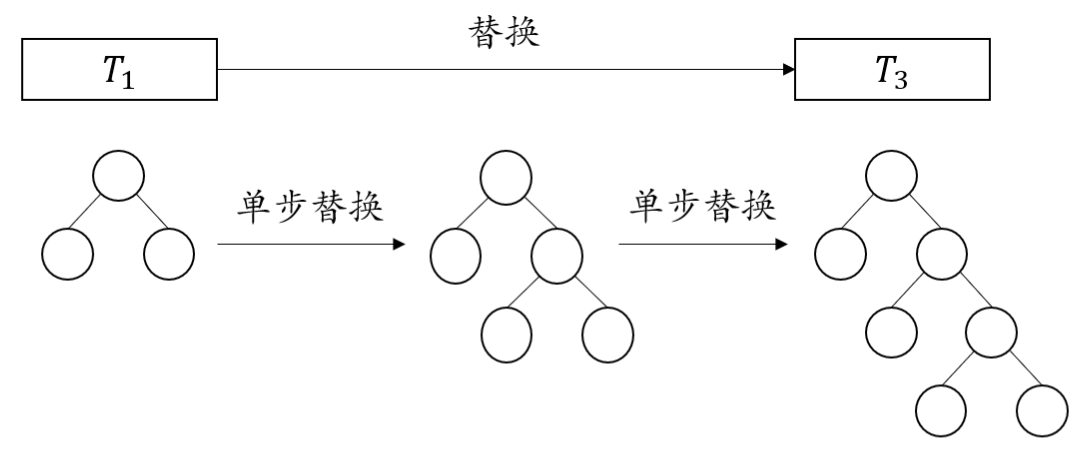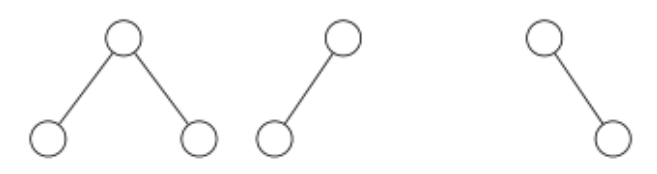# #562. 【NOI2020】超现实树

X2：省选前的集训真难熬啊……听课、考试、讲评、补题 —— 对于现在的我来说，即使在梦里想到一道数据结构题，也会不由自主地开始思考吧。

X1：重复训练对我来说似乎并不是什么负担，但我确实感觉到解决题目带来的愉悦感在最近逐渐减弱了。也许我们需要一些精神上的“刺激”：一些不拘泥于繁复技术的智力游戏，来让我们找回对于数学和算法的兴趣。

X2：咦，我好像收到了一封用英文写的短信，似乎是……数学书上的一些片段。

### 题目描述

X1：我来翻译一下短信的内容。

X2：也就是说,这里所说的树是指非空、有根、区分左右孩子的二叉树。

X1：的确如此。接下来书上定义了两棵树的同构。

1. 由单独结点构成的树是彼此同构的；
2. 如果两棵树的根结点均只有左子树，并且它们的左子树同构，那么这两棵树是同构的；
3. 如果两棵树的根结点均只有右子树，并且它们的右子树同构，那么这两棵树是同构的；
4. 如果两棵树的根结点均有左、右子树，并且它们的左、右子树分别对应同构，那么这两棵树是同构的。

X2：将同构的树看成相同的树就是说树的结点是彼此相同的。简单地说，两棵树同构当且仅当他们在结点无标号、区分左右孩子的意义下相同；我们说两棵树不同，当且仅当它们不同构。

X1：书里还定义了树的叶子：和通常的定义一样，叶子指没有任何孩子的结点

X2：这和我们熟悉的定义完全一致。嘛，数学家真是有点啰嗦……恐怕只有 X3 那种家伙会喜欢这种做派吧。

X1：我倒是对此不太反感——比起基于经验的“直觉”，准确的定义和严谨的证明还是更加让人安心。你看，下一个定义就没有那么直观了。

X2：我来想想……所谓替换，就是删掉某个叶子结点并在对应的位置放入另一棵树，就像那个叶子结点“长出了”一个更大的子树一样；一棵树替换成为另一棵树，说明它可以经由零次、一次或多次单步替换得到那棵树。哦……我明白了！举例来说，任何一棵树都可以替换成它本身，换言之对于树 $T$，都有 $T \to^{\star} T$。下面这个图片可以帮助理解单步替换和替换的含义。X1：你说得对。特别地，任何一棵树都可以替换得到无穷多棵不同的树，并且仅有一个结点构成的树可以替换得到任意其他的树。书上也有定义这样的东西。

X2：我们把 $\mathrm{grow}(\mathscr{T})$ 称作树的集合 $\mathscr{T}$ 所生长得到的集合吧 —— 也就是说，树的集合 $\mathscr{T}$ 所生长得到的集合包含所有可以被某个 $T \in \mathscr{T}$ 替换得到的树。不妨把树的集合叫做树林。不太严谨地说，一个树林所生长得到的新树林就是其中所有树、以所有可能的方式生长得到的树林。显而易见，一个非空树林所生长得到的树林都是无穷树林。但这个无穷树林，或者说 $\mathrm{grow}(\mathscr{T})$，并不一定包含所有的树 —— 更进一步,它甚至不一定包含“几乎所有”的树。

X1：让我来补充一下：我们称一个树林是几乎完备的（或称几乎包含了所有的树），如果仅有有限多的树不在其中。对于一个有限树林 $\mathscr{T}$ ，$\mathrm{grow}(\mathscr{T})$ 要么包含了所有的树，要么包含了几乎所有的树，要么存在无穷多棵树不在其中。如果这是一道 OI 题，出题人一定会在样例中给出三种情况的例子吧。书上的关键定理也用了和我们相同的定义。

X2：这个问题变成一个纯粹的 OI 题目了！让我用我们的语言来重述一下题意：给定一个有限大小的树林 $\mathscr{T}$，判定 $\mathrm{grow}(\mathscr{T})$ 是否是几乎完备的，即是否仅有有限棵树不能被树林中所包含的树生长得到

X1：也就是说，给定一个有限的树的集合 $\mathscr{T}$ ，判定是否仅有有限个树 $T$，满足 $T \notin \mathrm{grow}(\mathscr{T})$。所谓 $T \notin \mathrm{grow}(\mathscr{T})$，就是说不存在 $T' \in \mathscr{T}$，使得 $T' \to^{*} T$。这和通常的 OI 题目的确非常不同：我甚至没有想到这个问题的一个算法。

X2：我也一样，不过我很久没有感受到这种解决未知问题的冲动了。

### 输出格式

• 首先是一个正整数 $n$，表示树中的结点个数，结点编号为 $1,2,\cdots,n$；
• 接下来 $n$ 行每行两个非负整数，其中第 $i$ 行从左到右包含用空格隔开的 $l_i$ 和 $r_i$，分别表示 $i$ 号结点左、右孩子结点的编号。如果左（或右）孩子不存在，那么 $l_i$ （或 $r_i$）为 $0$。当然，叶结点一定满足 $l_i=r_i=0$。
• 输入数据保证构成一棵以 $1$ 号结点作为根结点的树。请注意：结点的编号只是为了方便输入，任何同构的树都被视为是相同的。

### 样例一

#### input

1
1
1
0 0


#### output

Almost Complete


### 样例二

#### input

1
3
3
2 3
0 0
0 0
2
2 0
0 0
2
0 2
0 0


#### output

Almost Complete


#### explanation### 样例三

#### input

1
2
3
2 3
0 0
0 0
2
2 0
0 0


#### output

No


### 数据范围

• 特殊性质 1：对于这一测试点中的每一组测试数据，都有 $m \le 4$，即树的集合中包括不超过 $4$ 棵树；
• 特殊性质 2：对于这一测试点中的每一组测试数据，树的集合中所有的树具有相同的高度；
• 特殊性质 3：对于这一测试点中的每一组测试数据，树的集合仅包含链（换言之，每个非叶结点仅包含一个孩子）；
• 特殊性质 4：对于这一测试点中的每一组测试数据，树的集合仅包含满足以下两个条件之一的树：
• 每个非叶结点仅包含一个孩子；
• 恰好有两个叶结点，它们具有相同的父结点，并且除这三个结点外，其余结点均有且仅有一个孩子。

1 $100$ $\le 1000$ $\le 1$
2 $\le 2$ 性质 1
3
4 $\le 1000000$ $\le 4$
5 $\le 5$ 性质 2
6 $\le 8$
7 $\le 9$ 性质 2
8 $\le 10$
9 $\le 1000000$ 性质 3
10 $20$ $\le 1000$ $\le 100$ $\le 1000$ 性质 4
11 $\le 2000$
12 $\le 100000$
13 $\le 200000$
14 $\le 800$ $\le 200$ $\le 800$
15 $\le 1000$ $\le 100$ $\le 1000$
16 $\le 2000$
17 $40$ $\le 300000$
18 $\le 600000$
19 $\le 900000$
20 $\le 1200000$
21 $\le 1500000$
22 $\le 2000000$
23
24
25